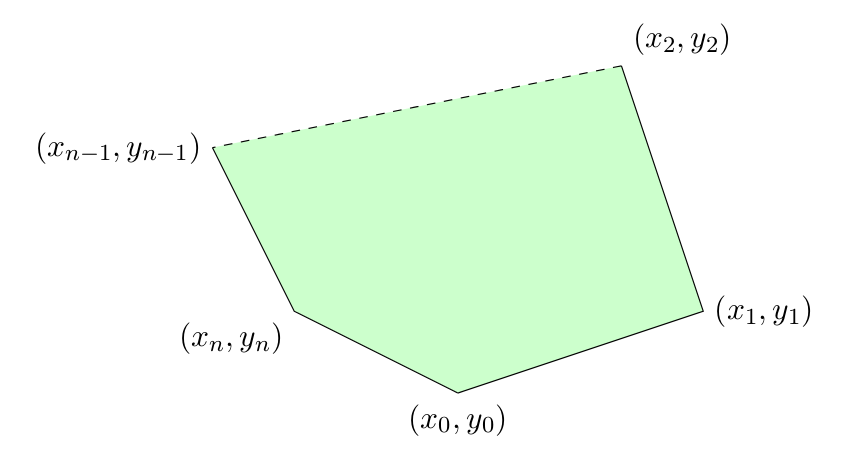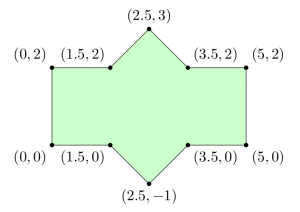﻿ Green’s Theorem and Area of Polygons « Stack Exchange Mathematics Blog

# Green’s Theorem and Area of Polygons

June 4, 2014 by . 9 comments

A common method used to find the area of a polygon is to break the polygon into smaller shapes of known area. For example, one can separate the polygon below into two triangles and a rectangle:By breaking this composite shape into smaller ones, the area is at hand: \begin{align}A_1 &= bh = 5\cdot 2 = 10 \\ A_2 = A_3 &= \frac{bh}{2} = \frac{2\cdot 1}{2} = 1 \\ A_{total} &= A_1+A_2+A_3 = 12\end{align}

Unfortunately, this approach can be difficult for a person to use when they cannot physically (or mentally) see the polygon, such as when a polygon is given as a list of many vertices.

## Formula

Happily, there is a formula for the area of any simple polygon that only requires knowledge of the coordinates of each vertex. It is as follows: $$A = \sum_{k=0}^{n} \frac{(x_{k+1} + x_k)(y_{k+1}-y_{k})}{2} \tag{1}$$ (Where $${n}$$ is the number of vertices, $${(x_k, y_k)}$$ is the $${k}$$-th point when labelled in a counter-clockwise manner, and $${(x_{n+1}, y_{n+1}) = (x_0, y_0)}$$; that is, the starting vertex is found both at the start and end of the list of vertices.)

It should be noted that the formula is not “symmetric” with respect to the signs of the $${x}$$ and $${y}$$ coordinates. This can be explained by considering the “negative areas” incurred when adding the signed areas of the triangles with vertices $${(0,0)-(x_k, y_k)-(x_{k+1}, y_{k+1})}$$.

In the next sections, I derive this formula using Green’s Theorem, show an example of its use, and provide some applications.

## Derivation

Green’s Theorem states that, for a “well-behaved” curve $${C}$$ forming the boundary of a region $${D}$$:

$$\displaystyle \oint_C P(x, y)\;\mathrm dx + Q(x, y)\;\mathrm dy = \iint_D \frac{\partial Q}{\partial x} – \frac{\partial P}{\partial y}\;\mathrm dA \ \ \ \ \ (2)$$

(In this context, “well behaved” means, among other things, that $${C}$$ is piecewise smooth. For a full listing of the requirements for $${C}$$ and $${D}$$, please see here.)

Recalling that the area of $${D}$$ is equal to $${\iint_D \;\mathrm dA}$$, we can use Green’s Theorem to calculate area if we choose $${P}$$ and $${Q}$$ such that $${\frac{\partial Q}{\partial x} – \frac{\partial P}{\partial y}=1}$$. Clearly, choosing $${P(x, y) = 0}$$ and $${Q(x, y) = x}$$ satisfies this requirement.

Thus, letting $${A}$$ be the area of the region $${D}$$:

$$\displaystyle A = \oint_C x\;\mathrm dy \ \ \ \ \ (3)$$

Now, consider the polygon below, bordered by the piecewise-smooth curve $${C = C_0\cup C_1\cup\cdots\cup C_{n-1}\cup C_n}$$, where $${C_k}$$ starts at the point $${(x_k, y_k)}$$ and ends at the “next” point along the polygon’s edge when proceeding counter-clockwise. (N.B. If we proceed in a clockwise manner, we get the negative of the area of the polygon.)Note: the dashed lines indicate that there could be any number of points between $${(x_2, y_2)}$$ and $${(x_{n-1}, y_{n-1})}$$; this is a complete polygon.

Because line integrals over piecewise-smooth curves are additive over length, we have that:

$$\displaystyle A = \oint_C x\;\mathrm dy = \int_{C_0} x\;\mathrm dy + \cdots + \int_{C_n} x\;\mathrm dy \ \ \ \ \ (4)$$

To compute the $${k}$$-th line line integral above, parametrize the segment from $${(x_k, y_k)}$$ to $${(x_{k+1}, y_{k+1})}$$:

$$\displaystyle C_k:\; \vec{r}=\left((x_{k+1} – x_k)t + x_k,\; (y_{k+1} – y_k)t + y_k\right),\quad 0\le t\le 1 \ \ \ \ \ (5)$$

Substituting this parametrization into the integral, we find: \begin{align}\int_{C_k} x\;\mathrm dy &= \int_0^1 \left((x_{k+1} – x_k)t + x_k\right)\left(y_{k+1} – y_k\right)\;\mathrm dt \\ &= \frac{(x_{k+1} + x_k)(y_{k+1}-y_{k})}{2}\end{align}

Summing all of the $$C_k$$’s, we then find the total area: $$A = \sum_{k=0}^{n} \frac{(x_{k+1} + x_k)(y_{k+1}-y_{k})}{2} \tag{6}$$

## Example

To demonstrate use of this formula, let us apply this to the shape at the beginning of the document. A copy of the image is found below, but this one is marked with the coordinates of the vertices.Starting with the coordinate $${(0, 0)}$$ and proceeding counter-clockwise (again, if we go in the opposite direction, we get the negative of the correct area), we apply our formula:

\begin{align} A &= \sum_{k=0}^{n} \frac{(x_{k+1} + x_k)(y_{k+1}-y_{k})}{2} \\ &= \frac{(1.5 + 0)(0 – 0)}{2} + \frac{(2.5 + 1.5)(-1 – 0)}{2} + \frac{(3.5 + 2.5)(0 – (-1))}{2} + \frac{(5+3.5)(0-0)}{2} \\ &\quad + \frac{(5+5)(2-0)}{2} + \frac{(3.5+5)(2-2)}{2} + \frac{(2.5+3.5)(3-2)}{2}\\ &\quad +\frac{(1.5+2.5)(2-3)}{2} + \frac{(0+1.5)(2-2)}{2} + \frac{(0+0)(0-2)}{2}\\ &= 0 + (-2) + 3 + 0 + 10 + 0 + 3 + (-2) + 0 + 0\\ &= 12 \end{align}

We arrive at the same answer we had above, which is good. (Obviously!)

## Possible Applications

Although this formula is an interesting application of Green’s Theorem in its own right, it is important to consider why it is useful. As can be seen above, this approach involves a lot of tedious arithmetic. Thus, its main benefit arises when applied in a computer program, when the tedium is no longer an issue.

From an engineering perspective, this formula could be used to determine the area (and, using similarly-derived formulas for the moments of a polygon about the $${x}$$ and $${y}$$ axes, the centroid) of component in a CAD program. Many computer programs estimate all shapes as polygons or connected line segments (rather than exact curves/circles), which means this formula can be directly applied to the existing representation in software.

In a contrived context, this formula can also be useful in collegiate programming competitions. (This was why I first took note of this formula.) Some problems in the “computational geometry” category may involve computing the area of specific polygons, such as the convex hull of a set of points. Knowing the derivation can make it easier to recall during a competition event.

As a final example, one can use this formula to approximate the area of a plot of land. Over a small region, surface area on a sphere can be approximated as that on a rectangle. Thus, an irregularly-shaped plot of land can be modelled as an polygon. Once coordinates are established for each vertex, the formula derived above can be applied to find its area.

•Steven Stadnicki says:

Not only can this formula be used to find areas, centroids, etc., but it regularly is; I’ve used it myself for determining the “best” location to place a letter in an arbitrarily-shaped polygon.

•Joel Reyes Noche says:

You say that there are $n$ vertices and your $k$ is from $0$ to $n$, so wouldn’t that involve $n+1$ vertices? Shouldn’t the first vertex be called $(x_1,y_1)$ and $k$ be from $1$ to $n$?

•Joel Reyes Noche says:

Also, in your example, shouldn’t the last term in the sum be (0+0)(0-2)/2?

•apnorton says:

The first point and the last point are the same. That, is $(x_0, y_0) = (x_n, y_n)$, So, although there are $n$ vertices, there are $n+1$ terms in the summation.

You are correct regarding the typo in the last term of the summation, though–I’ll change that.

•Interested Reader says:

So, how does this generalize to 3 or 4 dimensional polyhedra?

•Norbert says:

There is no natural order on the set of vertices of $n$ dimensional polyhedra. I think there is no good answer here.

•apnorton says:

I considered this, but didn’t make much progress. I believe that some similar formula for volume could be deduced through an application of Stoke’s Theorem, but I did not work on that long enough to produce anything of note.

•Jyrki Lahtonen says:

The problem of computing the volume of a 3D polyhedron given a list of its vertices as input is underspecified. Consider the following example. Start with a cube (8 vertices). Add a ninth vertex somewhere inside the cube. Remove from the cube a pyramid shaped part that has one of the faces of the original cube as its base and the freshly added ninth vertex as its peak. Depending on the choice of the face you get six different non-convex polyhedra, possibly all with distinct volumes (with a generically placed ninth vertex).

To describe the polyhedron you need to give its faces. Vertices alone won’t be enough.

•Thomas Klimpel says:

The list of vertices of a polygon can also be seen as the set of oriented boundary elements (=edge segments) of the polygon. So the analogy with the 3D polyhedron would be to compute the volume based on the set of oriented boundary elements (=faces) of the 3D polyhedron. But I think one has to use Gauss’ theorem (sometimes also called Divergence theorem or Ostrogradsky’s theorem), not the Kelvin–Stokes theorem.